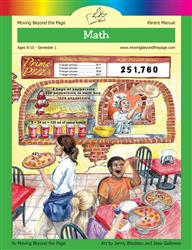Age 5-7 Reading Is Now Available!

# Common Core Alignment

CCSS.Math.Content.4.OA.4 - Find all factor pairs for a whole number in the range 1–100. Recognize that a whole number is a multiple of each of its factors. Determine whether a given whole number in the range 1–100 is a multiple of a given one-digit number. Determine whether a given whole number in the range 1–100 is prime or composite.

## 5: MathUnit 2: The Four Operations
Lesson 10: More Number Play
Lesson 14: Unit Test
Lesson 6: Multiples vs. Factors
Lesson 7: More Work With Factors
Lesson 8: Prime and Composite Numbers
Lesson 9: Extending Factors and Prime Numbers
Final Project: Different Types of NumbersUnit 4: Multi-Digit Multiplication
Lesson 1: Back to Multiplication Basics